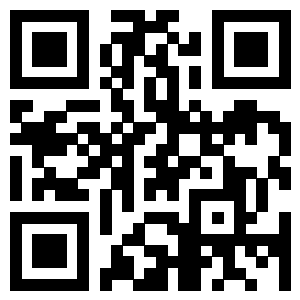# 海 南 靓 依 服 饰LIANGYI  FASHION  CO.LTD海南靓依服饰有限公司是海口一家资深高端制服

。欢迎有需要的朋友莅临指导。

，营造一种宾客至上的服务理念。

==================================================

<<<<<<<<<<<<<<<<<<<<<<<<<<<<<<<<<<<<<<<<<<<<<<<<<<行业新闻 职场搭配 制服常识

联系方式